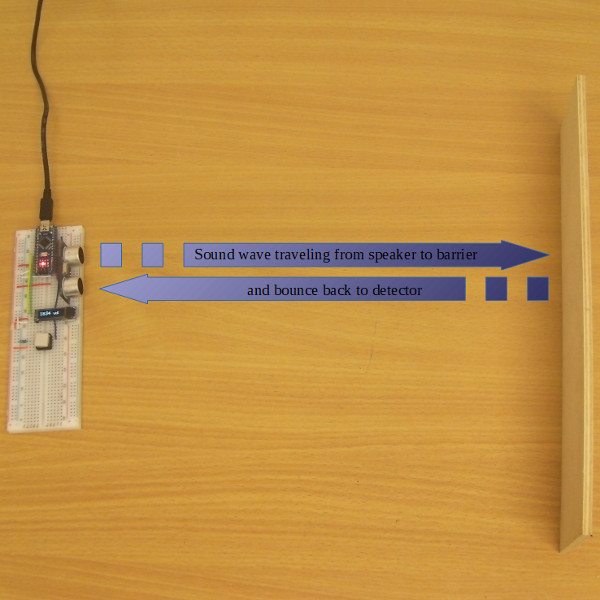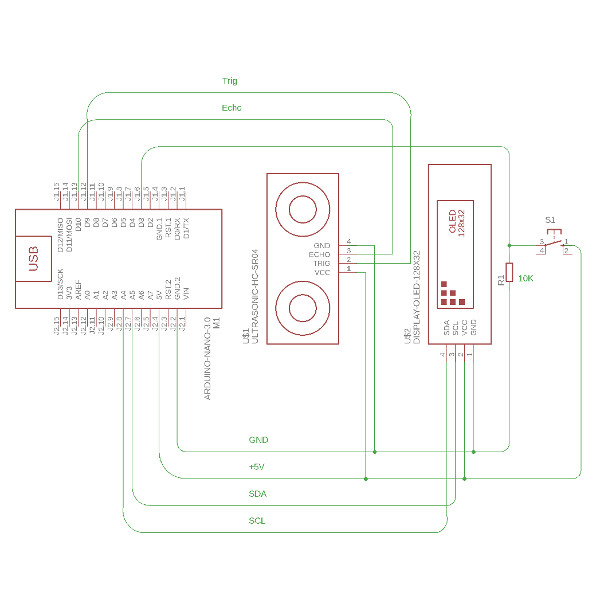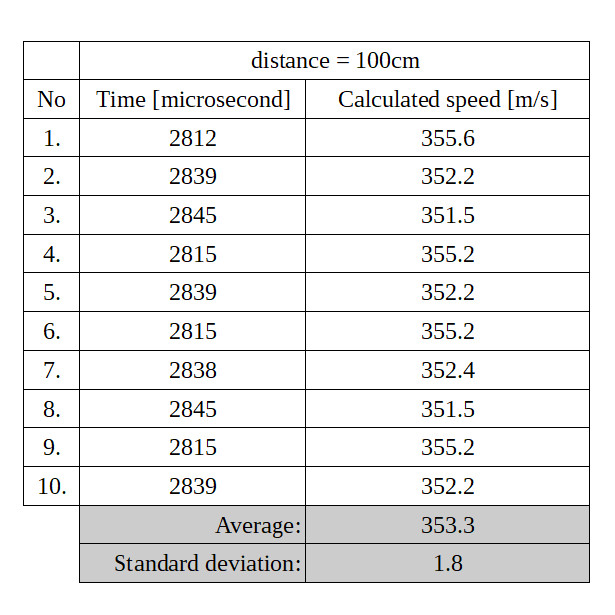Direct Measurements of the Speed of Sound
 The speed of sound is the distance travelled over time by a sound wave as it propagates through an elastic medium. In common everyday speech, the speed of sound refers to the speed of sound waves in air. [wikipedia]The HC-SR04 measures the time it takes for an emitted 40kHz ultrasound wave to travel to an obstacle and bounce back to the sensor. The time is measured in microseconds. Usually this is used to determine the distance to an object in a similar way to the one used by bats and dolphins. However, if the distance to the obstacle is already known, the HC-SR04 sensor can be used to determine the speed of sound. Our system contains an Arduino Nano, the HC-SR04 sensor, an 128x32 I2C OLED display with an SSD1306 driver, and a single button. The Arduino Nano communicates with the HC-SR04 module using two GPIO (D9 and D10)) and with the OLED display using an I2C bus. The time is measures with the pulseIn() function. The following libraries are used: Wire.h, Adafruit_SSD1306.h. The Arduino program can be downloaded here. The electronic scheme to the right was created in Autodesk Eagle ver 9.0.1 with the DIY Modules Library for EAGLEThe results of the speed of sound for distance equal to 100cm are presented in a table to the left. The average speed of sound is calculated in this experiment to be equal to 353.3m/s with the standard deviation being equal 1.8m/s. The speed of sound in air at 20°C is equal to 343m/s which makes our experimental error equal to 3%. We demonstrated a simple and inexpensive system which measured the speed of sound with reasonable precision.